## Reading the NSA’s codebaseLemonGraph review–Part VI–Executing queries

time to read 7 min | 1300 words

After going over many layers inside LemonGraph, I think we are ready to get to the real deal. We know how LemonGraph starts to execute a query. It find the clause that is likely to have the least number of items and starts from there. These are the seeds of the query, but how is it going to actually process that?

Here is my graph: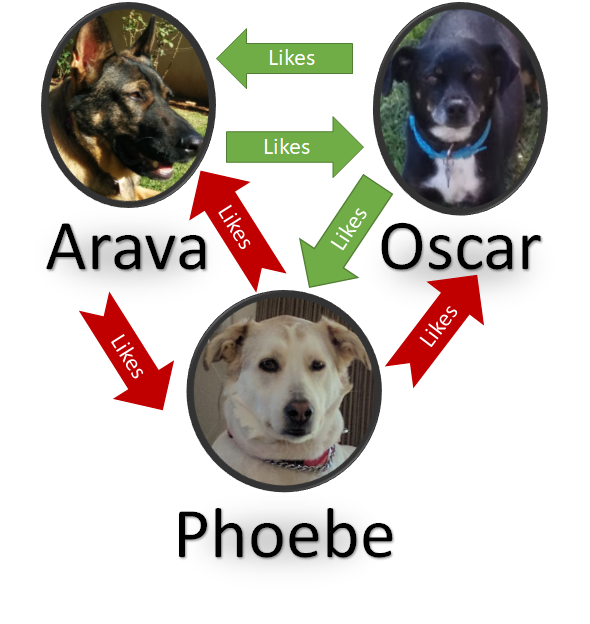And here is the query that I want to run on it: n()->e(type="likes", value="yes")->n()

LemonGraph detects that the cheapest source of seeds for this query is the edge and provides these to the MatchCTX class which does the actual processing. Let’s explore how it is working on a single seed.

The actual behavior starts from the matches() method, which starts here: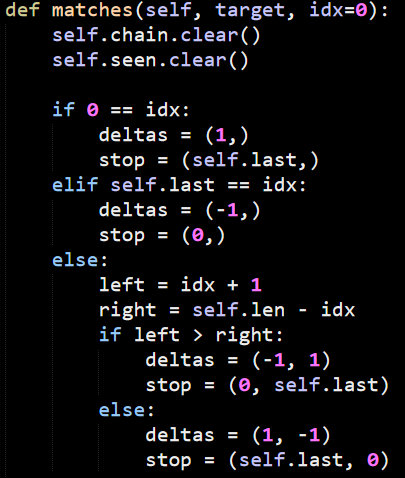As usual for this codebase, the use of tuples for controlling behavior is prevalent and annoying. In this case, the idx argument controls the position of the target in the query, whatever this is the start, end or somewhere in the middle. The deltas define what direction the matches should go and the stops where you must stop searching, I think.

Now that we setup the ground rules, the execution is as follows: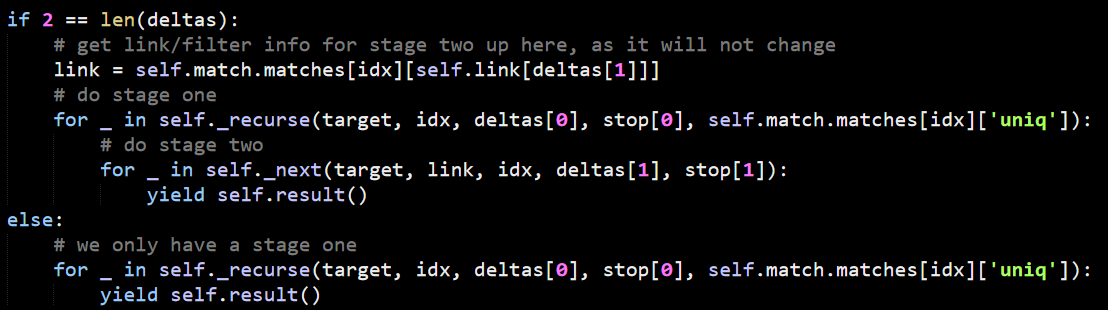In this case, because the seed of our query is the edge (which is in the middle) it determines that deltas are (1, –1) and stops are (2, 0). We’ll also go to the first clause in the if statement. The link variable there controls whatever we should follow links going in or out.

There is a lot of logic actually packed into the link initialization. The self.link is an array that has ‘next’ and ‘prev’ as its values, so the idea is that it find what property to look at, then use the dictionary syntax to get the relevant property and decide what kind of direction it should go.

We’ll focus on the _recurse() method for now. In this case, we are being passed:

• idx = 1
• delta = 1
• stop = 2

Here is the actual method:As you can see, we first validate that the match is valid (this is where we mostly check other properties of the value that we are currently checking, filtering them in place). Usually the do_seen will be set to True, which will ensure that we only traverse each node once. The main iteration logic is done in combination with the _next() method, shown below: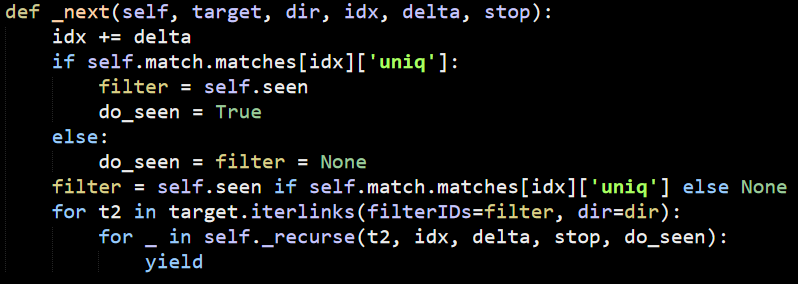The first line there shows usage of delta, which control in what direction to move. I’m not quite sure why the filter is set in the if statements, since it is also being set immediately after. This looks like redundant code that snuck in somehow.

The bulk of the work is shelled out to iterlinks, so let’s check what is going on there… I know that we are running on an edge here, so we need to look at the Edge.iterlinks() method: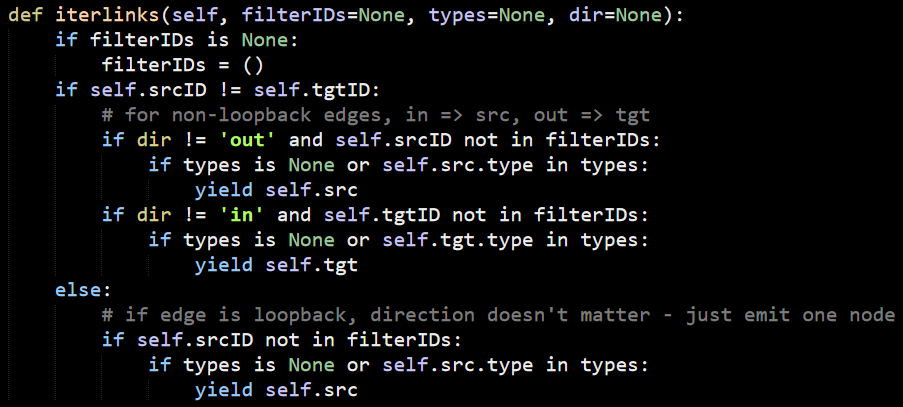There isn’t really much to tell here, it checks the filters and return the incoming or outgoing edges, nothing more. On the other hand, the Node.iterlinks() implementation is a bit simpler: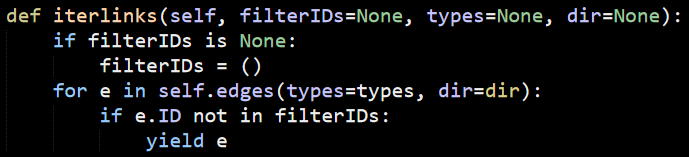We’ll explore exactly how the edges are loaded for a node in a bit, however, I do want to note right now that the _next() method isn’t passing the types of the edge, even though it looks to me that it has this information. In that case, that would give a performance boost because it could filter a lot of edges in busy graphs.

Actually, I already looked at how iterators working in a previous post, so I won’t go over all of that again. This is basically calling this method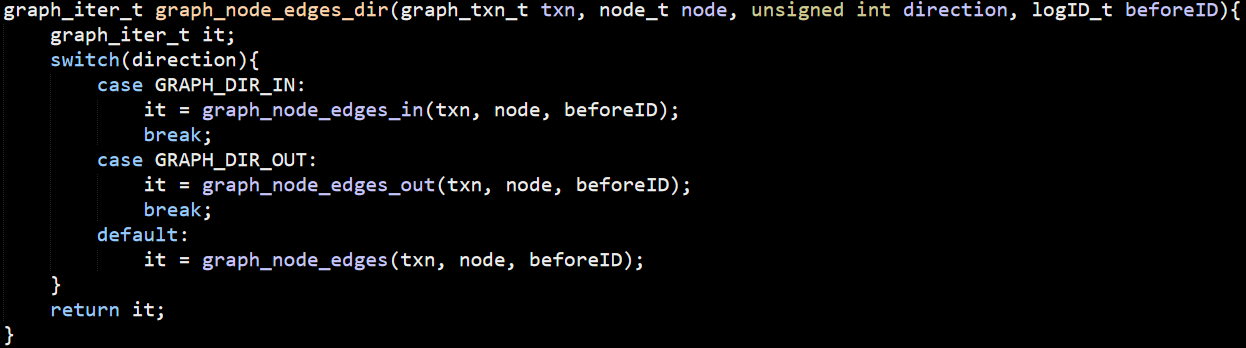The graph_node_edges_in() and graph_node_edges_out() methods are pretty simple, basically just scanning the DB_TGTNODE_IDX or DB_SRCNODE_IDX indexes, respectively. The graph_node_edges() however, is really strange.

Here it is: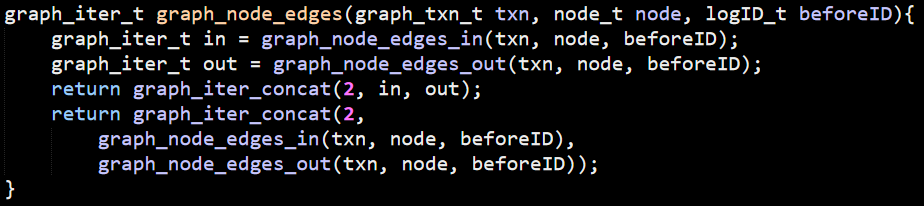It took me a few reads to figure out that this is probably a case of someone unpacking a statement for debugging but forgetting to remove the packed statement. The second return statement is ignored and likely stripped out as unreachable, but it is pretty confusing to read.

The graph_iter_concat() answers a question I had about how graph_iter_t is used, here is how it looks like:This is using C’s support for passing an unknown number of parameters to a method. This basically builds a simple linked list, which also answers the usage of _blarf() function and its behavior a few posts ago.

So we are back were we started, we understand how the data flows into the Python code, now let’s go back and look at _recurse() and _next() calls.

Now I know a lot more about the behavior of the code, so this make sense. The stop argument to the _recurse() control the depth of the number of links that would be traversed in a particular query. Now that I know how this particular clause work, I understand how LemonGraph goes from the edge to the node in e()->n(), but I don’t know how it goes to back to find the full path.

The key for that are the calls to push()  and pop() in the the MatchCtx._recurse() methods. These update the chain, like so: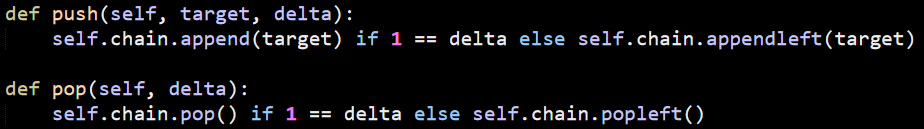In processing the query, we first append the edge to the chain:The next step goes from the edge to it’s destination, giving us Oscar:Remember that in the matches(), we got into this if clause:This is when we start scanning an edge, which first add things to the right, then it scan things to the left in the _next() method. Look at the push() method there. By the time we get to the result(), we have iterated both sides of the connection, giving us: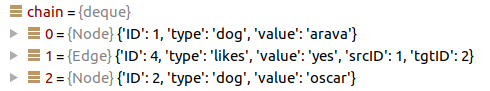That is a very clever way of doing things.

Let’s try doing things a little bit differently. I’m going to check a slightly different query: n(type=”dog”, value=”arava”)->e(type="likes", value="yes")->n()

If I understand things correctly, this is going to select the node as the starting point, because that is a lot more efficient. That will allow me to figure out the other side of this operation. I just run this through the debugger, and this is indeed how it actually works.

The usage of yield to pass control midway is not trivial to follow, but it ends up being quite a nice implementation. This is enough for this post. In my next one, I want to explore a few of the possible operations that exposed by LemonGraph.